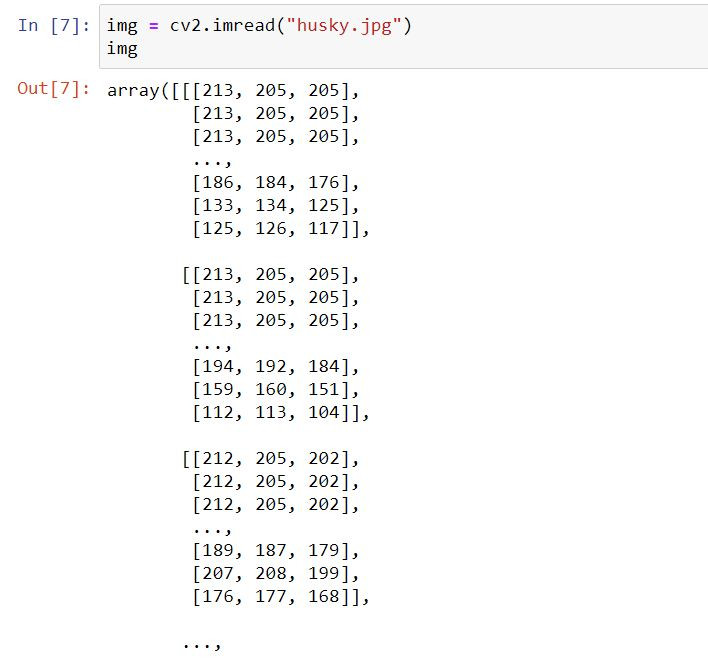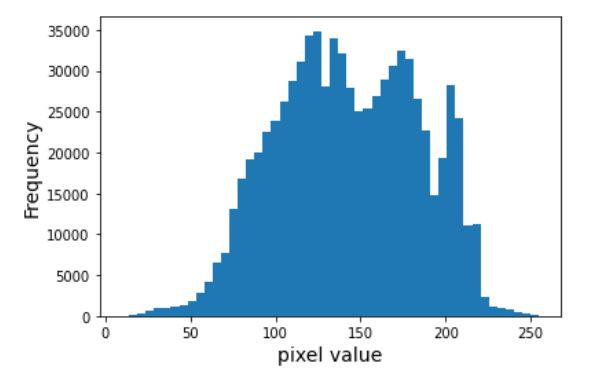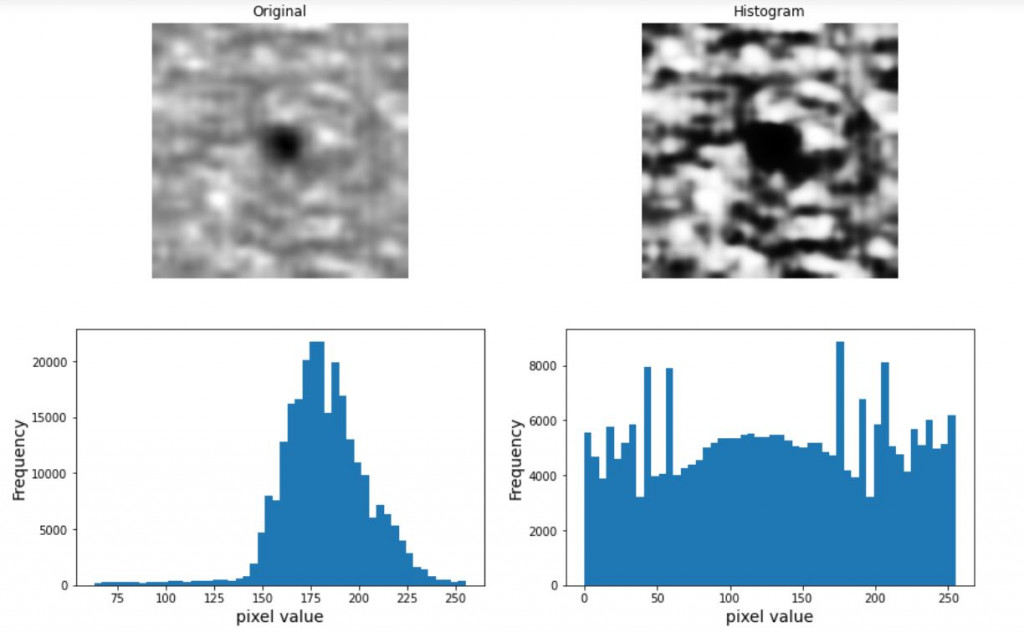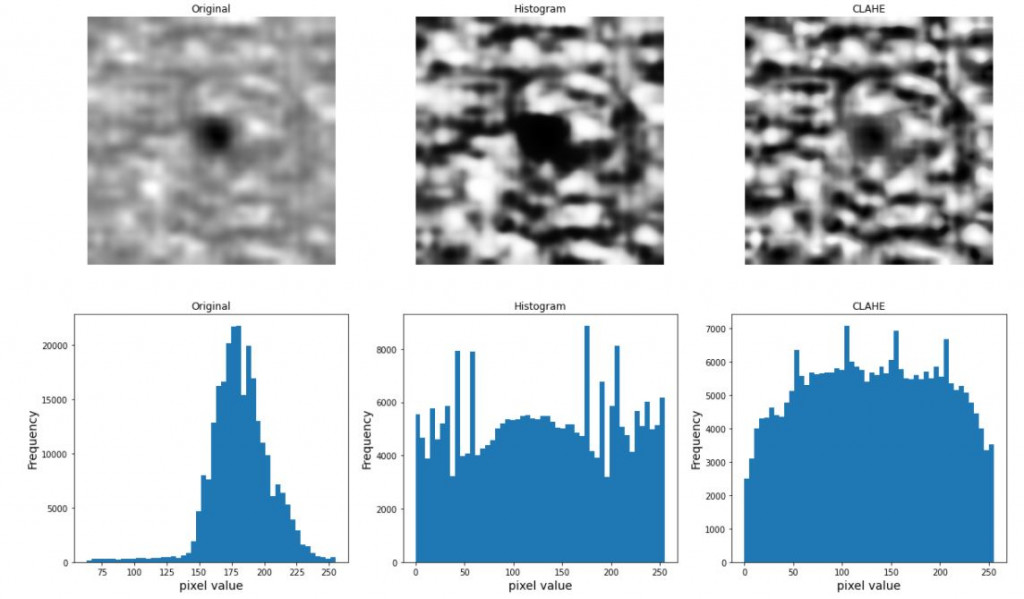#DAY 20
0
AI & Data

## 一、圖片組成

Size：圖片的大小，圖片是二維的，大小的表示就是Length*Width
Channel：通道數，簡單來說就是幾種基本顏色組成的，一般的彩色照片通道數就是3(RGB)
Shape： 圖片的完整信息，包括Size及Channel數，EX: (Length,Width,Channel)

## 二、處理套件-OpenCV

OpenCV是目前相當廣泛的圖片處理套件，它包括簡單的圖片基本處理，也有較進階的使用方法，因為很多人使用，因此在網路上也找的到很多資源，相當方便，下面就帶大家進行一些簡單的處理。

### 要使用此套件前需先下載資源包(Anaconda)

``````conda install opencv
``````

### 載入套件

``````import cv2  #圖片處理
import matplotlib.pyplot as plt  #畫出圖片
``````

### 讀取圖片，這邊可以看到讀進來的圖片會以陣列顯示像素

``````img = cv2.imread("husky.jpg")
img
``````### 顯示圖片

``````plt.imshow(img,cmap=None) #參數cmap是輸出照片的顏色，如果是黑白照片要設為灰階圖"gray"
``````### 查看圖片資訊(shape,資料型態,最大最小像素值)

``````print(f"image shape: {img.shape}")
print(f"data type: {img.dtype}")
print(f"min: {img.min()}, max: {img.max()}")
``````### 縮放圖片

``````img=cv2.resize(img,(512,512))
``````

## 三、進階圖片處理

### 直方圖均衡化(Histogram Equalization)

``````plt.hist(img.reshape(-1), bins=50)
plt.xlabel("pixel value", fontsize=14)
plt.ylabel("Frequency", fontsize=14)
``````### 這邊我們以一張灰階圖做示範

``````#Histogram Equalization
equalize_img = cv2.equalizeHist(img)

plt.figure(figsize=(14, 9))
plt.subplot(2, 2, 1)
plt.imshow(img, cmap="gray")
plt.axis("off")
plt.title(f"Original")

plt.subplot(2, 2, 2)
plt.imshow(equalize_img, cmap="gray")
plt.axis("off")
plt.title(f"Histogram")

plt.subplot(2, 2, 3)
plt.hist(img.reshape(-1), bins=50)
plt.xlabel("pixel value", fontsize=14)
plt.ylabel("Frequency", fontsize=14)

plt.subplot(2, 2, 4)
plt.hist(equalize_img.reshape(-1), bins=50)
plt.xlabel("pixel value", fontsize=14)
plt.ylabel("Frequency", fontsize=14)
plt.show()
``````### 局部均衡化(createCLAHE)

``````clahe = cv2.createCLAHE()
clahe_img = clahe.apply(img)

plt.figure(figsize=(20, 12))
plt.subplot(2, 3, 1)
plt.imshow(img, cmap="gray")
plt.axis("off")
plt.title(f"Original")

plt.subplot(2, 3, 2)
plt.imshow(equalize_img, cmap="gray")
plt.axis("off")
plt.title(f"Histogram")

plt.subplot(2, 3, 3)
plt.imshow(clahe_img, cmap="gray")
plt.axis("off")
plt.title(f"CLAHE")

plt.subplot(2, 3, 4)
plt.hist(img.reshape(-1), bins=50)
plt.xlabel("pixel value", fontsize=14)
plt.ylabel("Frequency", fontsize=14)
plt.title(f"Original")

plt.subplot(2, 3, 5)
plt.hist(equalize_img.reshape(-1), bins=50)
plt.xlabel("pixel value", fontsize=14)
plt.ylabel("Frequency", fontsize=14)
plt.title(f"Histogram")

plt.subplot(2, 3, 6)
plt.hist(clahe_img.reshape(-1), bins=50)
plt.xlabel("pixel value", fontsize=14)
plt.ylabel("Frequency", fontsize=14)
plt.title(f"CLAHE")
plt.show()
``````